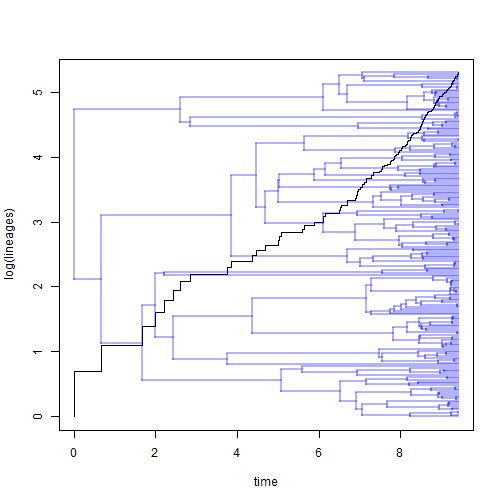## Monday, October 23, 2017

### Birth-death model-fitting using phytools

The following is some code to compute the likelihood & to fit a simple birth-death model to the branching times on a phylogeny, allowing an incomplete sampling fraction.

The likelihood function is based on Stadler (2012). Thanks to Luke Harmon for pointing me in the right direction for this.

This doesn't do anything not already available in R, but my intention is to use it to make a `ratebytree` function for comparing the diversification rates (speciation &/or extinction) between trees. In fact, I'm going to check it against `birthdeath` in ape and `make.bd` in diversitree to verify that it is working correctly.

First, here are the functions I will use:

``````lik.bd<-function(theta,t,rho=1,N=NULL){
lam<-theta
mu<-theta
if(is.null(N)) N<-length(t)+1
p0ti<-function(rho,lam,mu,t)
1-rho*(lam-mu)/(rho*lam+(lam*(1-rho)-mu)*exp(-(lam-mu)*t))
p1ti<-function(rho,lam,mu,t)
rho*(lam-mu)^2*exp(-(lam-mu)*t)/(rho*lam+(lam*(1-rho)-mu)*exp(-(lam-mu)*t))^2
lik<-2*log(p1ti(rho,lam,mu,t))-2*log(1-p0ti(rho,lam,mu,t))
for(i in 2:length(t)) lik<-lik+log(lam)+log(p1ti(rho,lam,mu,t[i]))
-(lik+lfactorial(N-1))
}

fit.bd<-function(tree,b=NULL,d=NULL,rho=1,...){
init<-vector(length=2)
if(hasArg(init.b)) init<-init.b
else init<-(log(Ntip(tree))-log(2))/max(nodeHeights(tree))
if(hasArg(init.d)) init<-init.d
else init<-0
if(!is.binary(tree)) tree<-multi2di(tree)
T<-sort(branching.times(tree),decreasing=TRUE)
fit<-optim(init,lik.bd,t=T,rho=rho,method="L-BFGS-B",lower=rep(0,2),
upper=rep(Inf,2))
obj<-list(b=fit\$par,d=fit\$par,rho=rho,logL=-fit\$value,
opt=list(counts=fit\$counts,convergence=fit\$convergence,
message=fit\$message))
class(obj)<-"fit.bd"
obj
}

print.fit.bd<-function(x, ...){
if(hasArg(digits)) digits<-list(...)\$digits
else digits<-4
cat("\nFitted birth-death model:\n\n")
cat(paste("ML(b/lambda) =",round(x\$b,digits),"\n"))
cat(paste("ML(d/mu) =",round(x\$d,digits),"\n"))
cat(paste("log(L) =",round(x\$logL,digits),"\n"))
cat(paste("\nAssumed sampling fraction (rho) =",x\$rho,"\n"))
if(x\$opt\$convergence==0) cat("\nR thinks it has converged.\n\n")
else cat("\nR thinks optimization may not have converged.\n\n")
}
``````

Next, let's simulate a tree:

``````library(phytools)
tree<-pbtree(n=200,b=1.0,d=0.5,extant.only=TRUE)
tree
``````
``````##
## Phylogenetic tree with 200 tips and 199 internal nodes.
##
## Tip labels:
##  t27, t140, t195, t196, t155, t156, ...
##
## Rooted; includes branch lengths.
``````
``````ltt(tree,show.tree=TRUE)
````````````## Object of class "ltt" containing:
##
## (1) A phylogenetic tree with 200 tips and 199 internal nodes.
##
## (2) Vectors containing the number of lineages (ltt) and branching times (times) on the tree.
##
## (3) A value for Pybus & Harvey's "gamma" statistic of 4.1273, p-value = 0.
``````

Now let's fit our model:

``````fit<-fit.bd(tree)
fit
``````
``````##
## Fitted birth-death model:
##
## ML(b/lambda) = 1.1156
## ML(d/mu) = 0.7328
## log(L) = 597.2846
##
## Assumed sampling fraction (rho) = 1
##
## R thinks it has converged.
``````

Let's check if this matches ape & diversitree:

``````birthdeath(tree) ## likelihood the same
``````
``````##
## Estimation of Speciation and Extinction Rates
##             with Birth-Death Models
##
##      Phylogenetic tree: tree
##         Number of tips: 200
##               Deviance: -1194.569
##         Log-likelihood: 597.2846
##    Parameter estimates:
##       d / b = 0.6568466   StdErr = 0.07194047
##       b - d = 0.3828361   StdErr = 0.05980628
##    (b: speciation rate, d: extinction rate)
##    Profile likelihood 95% confidence intervals:
##       d / b: [0.5647784, 0.7295066]
##       b - d: [0.3196238, 0.4553609]
``````
``````bd(birthdeath(tree)) ## estimates the same
``````
``````##         b         d
## 1.1156412 0.7328051
``````
``````library(diversitree)
lik<-make.bd(tree)
find.mle(lik,c(1,0))
``````
``````## \$par
##    lambda        mu
## 1.1156350 0.7327982
##
## \$lnLik
##  597.2846
##
## \$counts
##  8
##
## \$code
##  1
##
##  -0.0005105353  0.0003506102
##
## \$method
##  "nlm"
##
## \$func.class
##  "bd"       "dtlik"    "function"
##
## attr(,"func")
## Constant rate birth-death likelihood function:
##   * Parameter vector takes 2 elements:
##      - lambda, mu
##   * Function takes arguments (with defaults)
##      - pars: Parameter vector
##      - condition.surv [TRUE]: Condition likelihood on survial?
##      - intermediates [FALSE]: Also return intermediate values?
##   * Phylogeny with 200 tips and 199 nodes
##      - Taxa: t27, t140, t195, t196, t155, t156, t124, t127, ...
##   * Reference:
##      - Nee et al. (1994) doi:10.1098/rstb.1994.0068
## R definition:
## function (pars, condition.surv = TRUE, intermediates = FALSE)
## attr(,"class")
##  "fit.mle.bd" "fit.mle"
``````

Looks good so far. Now let's try with an incomplete sampling fraction:

``````tree50<-drop.tip(tree,sample(tree\$tip.label,round(0.5*Ntip(tree))))
tree50
``````
``````##
## Phylogenetic tree with 100 tips and 99 internal nodes.
##
## Tip labels:
##  t195, t155, t156, t124, t127, t128, ...
##
## Rooted; includes branch lengths.
``````
``````fit.bd(tree50,rho=0.5)
``````
``````##
## Fitted birth-death model:
##
## ML(b/lambda) = 1.155
## ML(d/mu) = 0.7603
## log(L) = 190.816
##
## Assumed sampling fraction (rho) = 0.5
##
## R thinks it has converged.
``````
``````lik<-make.bd(tree50,sampling.f=0.5)
find.mle(lik,c(1,0.5))
``````
``````## \$par
##    lambda        mu
## 1.1549530 0.7603125
##
## \$lnLik
##  190.816
##
## \$counts
##  7
##
## \$code
##  1
##
##   4.806048e-05 -5.209699e-05
##
## \$method
##  "nlm"
##
## \$func.class
##  "bd"       "dtlik"    "function"
##
## attr(,"func")
## Constant rate birth-death likelihood function:
##   * Parameter vector takes 2 elements:
##      - lambda, mu
##   * Function takes arguments (with defaults)
##      - pars: Parameter vector
##      - condition.surv [TRUE]: Condition likelihood on survial?
##      - intermediates [FALSE]: Also return intermediate values?
##   * Phylogeny with 100 tips and 99 nodes
##      - Taxa: t195, t155, t156, t124, t127, t128, t81, t98, ...
##   * Reference:
##      - Nee et al. (1994) doi:10.1098/rstb.1994.0068
## R definition:
## function (pars, condition.surv = TRUE, intermediates = FALSE)
## attr(,"class")
##  "fit.mle.bd" "fit.mle"
``````

Neat. It seems to work.